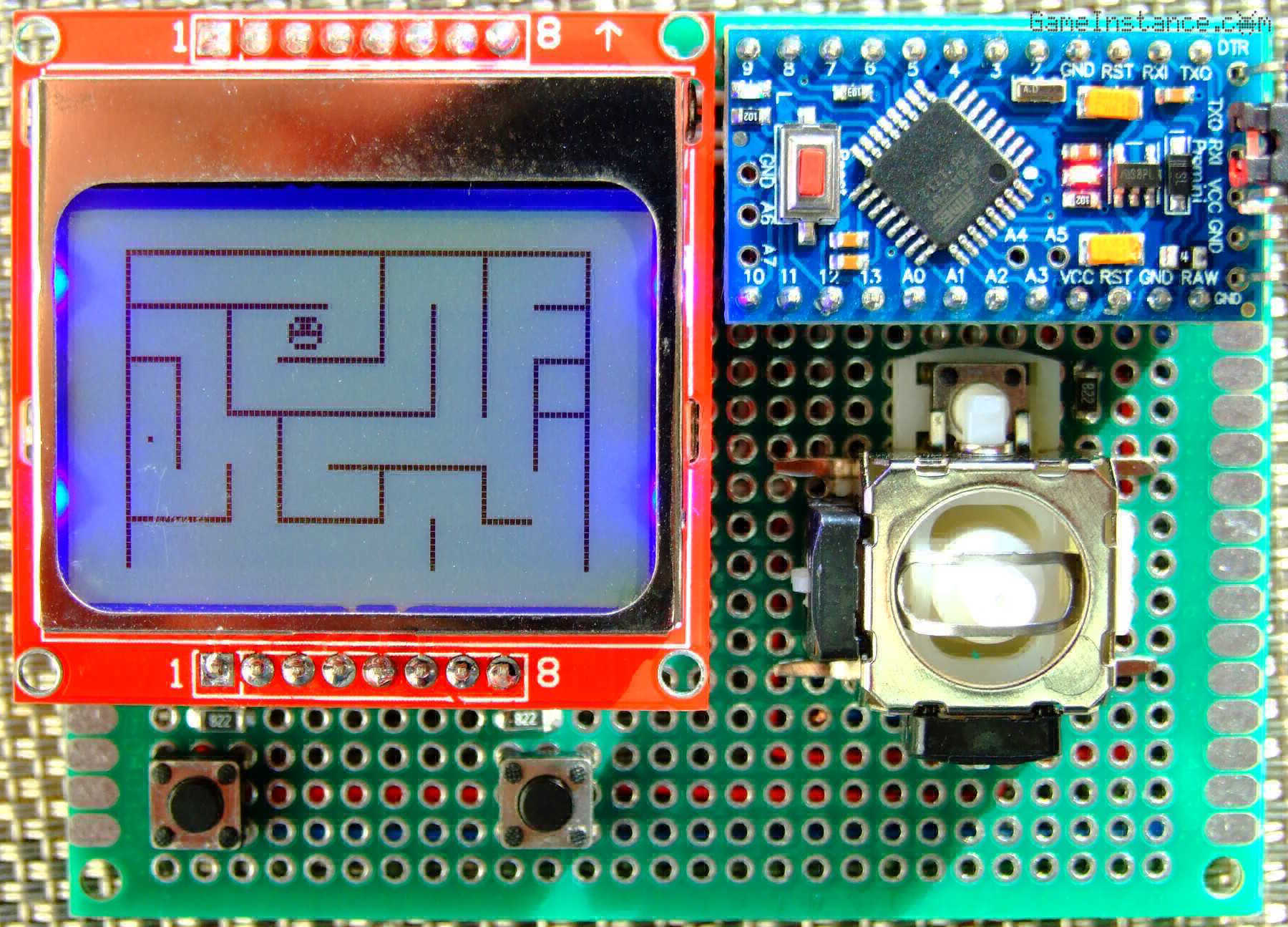# Game Instance

Let the games begin

After the Space Invaders replica, it is now time for a new Arduino Console app, in this case a maze exploring game. To play it, you'll have to move the character within the maze looking for the exit point marked with, well, a point.Maze Explorer game - on the first revision of the Arduino Game Console

### The code

centers around the MazeExplorer class, a GameConsole extension that mainly overrides the Execute method. To reserve its place in the EEPROM memory it also defines a GameIndex. The finite state machine has only 8 states for initialization, controls reading, movement, display, game complete test, waiting state and a few others of less importance.

The character can travel from one fixed position to another either up, down, left or right according to the permissions and restrictions of the maze. Each such maze position is represented using two bits, for left and up restrictions.Maze position restrictions: 0 = none, 1 = up is blocked, 2 = left is blocked, 3 = both are blocked

There's no need to store restrictions for the other two directions because there's always a position to the right or down that can do that. Considering a rectangular shaped maze, the absolute edges can be determined and drawn accordingly. As such, four maze positions can be stored using one byte, considerably reducing the memory footprint. That helps a lot given Atmega328's RAM size.

```#include <GameConsole.h>

struct Point {

unsigned char m_x, m_y;

void Init(unsigned char x, unsigned char y) {
//
m_x = x;
m_y = y;
}
};

struct Maze {

// can travel up
bool UpBlocked(unsigned int i) {
//
if (PLAIN) {
//
return (m_map[i] & 0x01);
}
return (m_map[i / 4] & (0x01 << ((3 - (i % 4)) * 2)));
}
// can travel left
bool LeftBlocked(unsigned int i) {
//
if (PLAIN) {
//
return (m_map[i] & 0x02);
}
return (m_map[i / 4] & (0x02 << ((3 - (i % 4)) * 2)));
}

/// maze size
static const unsigned char W = 9, H = 6;
/// the maze
unsigned char m_map[W * H] {
3, 1, 1, 1, 1, 3, 1, 3, 1,
3, 1, 3, 1, 0, 2, 2, 2, 1,
3, 2, 2, 1, 1, 0, 2, 2, 3,
2, 2, 1, 3, 1, 1, 0, 0, 3,
2, 0, 2, 2, 1, 3, 1, 2, 0,
3, 1, 0, 1, 1, 0, 2, 1, 0,
};
/// plain data representation
static const bool PLAIN = true;
};

struct Walker : public Point {

static const byte TICKNESS = 6;

unsigned char m_direction;
signed char m_dx, m_dy;

Walker() : Point(),
m_direction(0),
m_dx(0), m_dy(0) {
//
}

/// still moving
bool IsMoving() {
//
return (m_direction != 0);
}
/// goes horizontally
void GoX(signed char x, const Maze& maze) {
//
if ((m_x + maze.H < 0) || (m_x + x > maze.W - 1)) {
//
return;
}
if (x < 0) {
// go left
if (maze.LeftBlocked(m_x + m_y * maze.W)) {
// left is blocked
return;
}
m_direction = 1;
return;
}
if (x > 0) {
// go right
if (maze.LeftBlocked(m_x + x + m_y * maze.W)) {
// right is blocked
return;
}
m_direction = 3;
return;
}
return;
}
/// goes vertically
bool GoY(signed char y, const Maze& maze) {
//
if ((m_y + y < 0) || (m_y + y > maze.H - 1)) {
//
return;
}
if (y < 0) {
// go up
if (maze.UpBlocked(m_x + m_y * maze.W)) {
// up is blocked
return;
}
m_direction = 2;
return;
}
if (y > 0) {
// go down
if (maze.UpBlocked(m_x + (m_y + y) * maze.W)) {
// down is blocked
return;
}
m_direction = 4;
return;
}
return;
}
/// moves the walker
bool Move(unsigned char width, unsigned char height) {
//
if (m_direction == 1) {
// going left
m_dx -= 1;
if (m_dx <= -width) {
//
m_dx = 0;
m_x -= 1;
m_direction = 0;
}
}
if (m_direction == 2) {
// going up
m_dy -= 1;
if (m_dy <= -height) {
//
m_dy = 0;
m_y -= 1;
m_direction = 0;
}
}
if (m_direction == 3) {
// going right
m_dx += 1;
if (m_dx >= width) {
//
m_dx = 0;
m_x += 1;
m_direction = 0;
}
}
if (m_direction == 4) {
// going down
m_dy += 1;
if (m_dy >= height) {
//
m_dy = 0;
m_y += 1;
m_direction = 0;
}
}
}
};

const unsigned char Walker::MASK = {0x0E, 0x15, 0x17};

class MazeExplorer : public GameConsole {

// CODE REMOVED
// Check-out https://github.com/gameinstance/game-console-1/
};

/// the game instance
MazeExplorer game;

void setup() {
// put your setup code here, to run once:
game.Setup();
}

void loop() {
// put your main code here, to run repeatedly:
game.Loop();
}```

Do check out the github repository for the complete, up-to-date code.

### Where

can we go from here? Well, the so-called game can be improved by adding enemy creatures wandering about the maze, making life difficult, leading to a Pac-man like experience. It can further be extended by allowing the use of weapons, turning it into Bomberman. The possibilities are limited by the hardware and one's imagination.